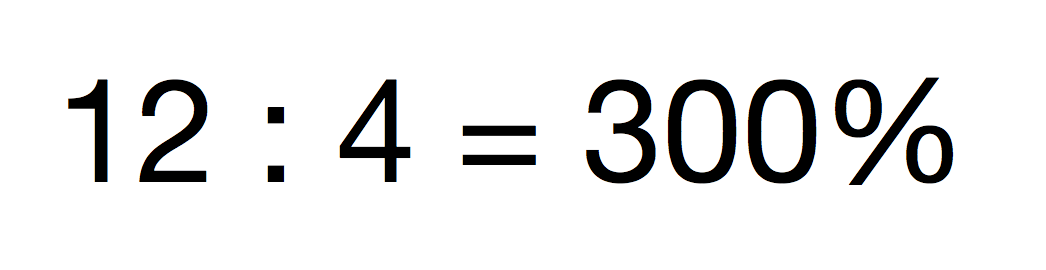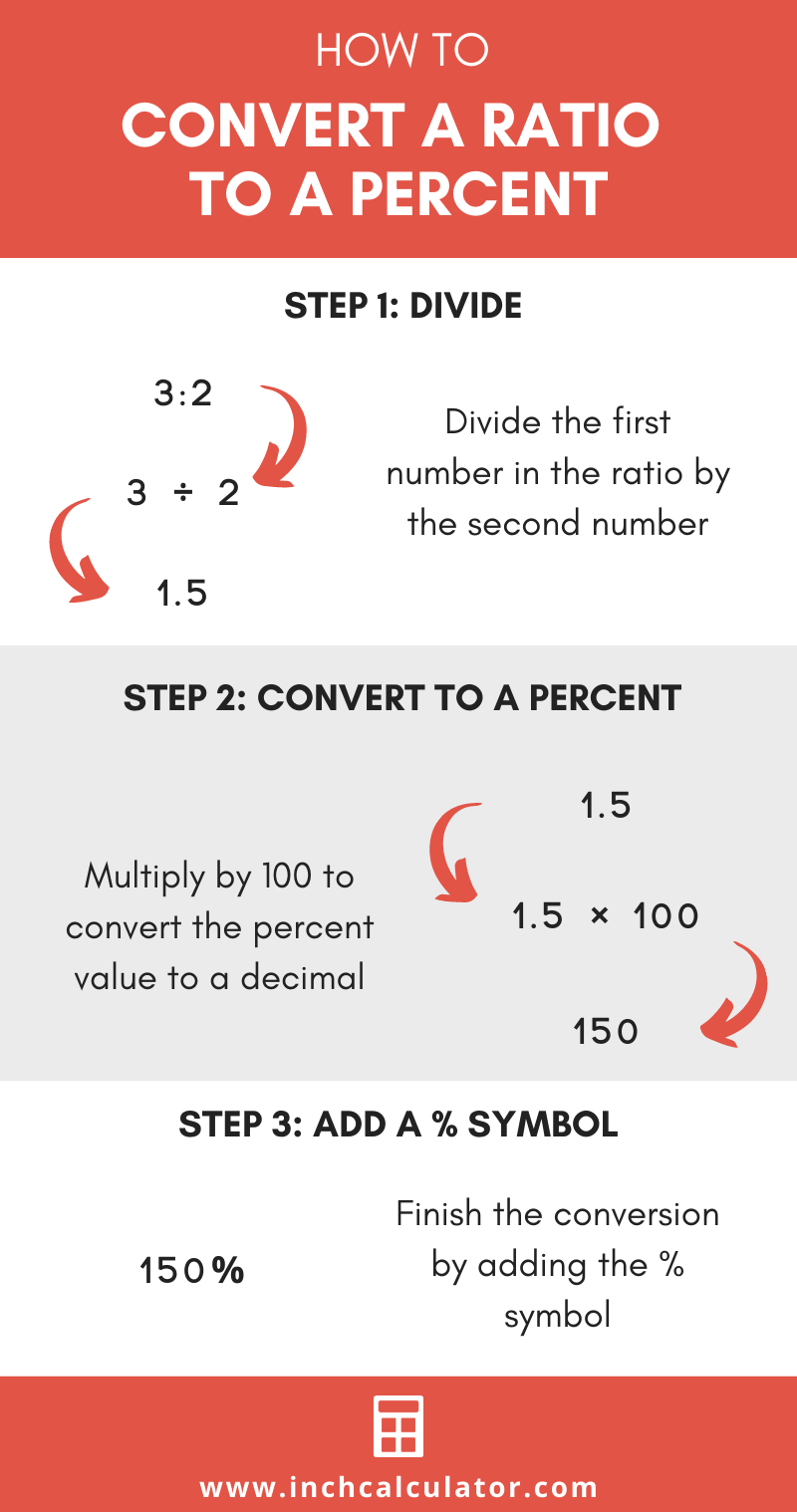# Ratio to Percentage Calculator

Convert a ratio into a percentage by entering the ratio in the fields below.

Ratio
:

## Percentage:

150%
Learn how we calculated this below

## How to Convert a Ratio to a Percentage

Ratios are often expressed in the form m:n or m/n. To convert a ratio into the form of a percentage, simply divide m by n and then multiply the result by 100.

### Steps to Convert

The conversion consists of a few simple steps:

1. Step 1: Convert the ratio into a fraction
2. Step 2: Divide the first number by the second number
3. Step 3: Multiply by 100 to convert to a percentage
4. Step 4: Add the percent symbol (%)

For example, If the ratio is 12:4, convert it to the form 12/4, which is an equation we can solve. After that, multiply the result by 100 to get the percentage.

12 ÷ 4 = 3
3 × 100 = 300%Use our ratio calculator to solve or reduce a ratio.